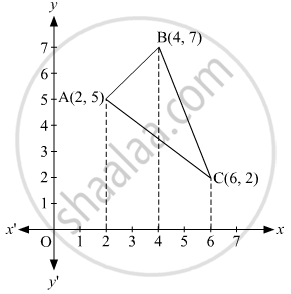# Using Integration, Find the Area of Triangle Abc, Whose Vertices Are A(2, 5), B(4, 7) and C(6, 2). - Mathematics

Sum

Using integration, find the area of triangle ABC, whose vertices are A(2, 5), B(4, 7) and C(6, 2).

#### SolutionVertices of the given triangle are A(2,5), B(4,7), and C(6,2).
Equation of AB
y - 5 = (7-5)/(4-2) (x -2)
⇒ y - 5 = x -2
⇒  y = x + 3

Let's say y1= x+3

Equation of BC:
y -7= (2-7)/(6-4)(x-4)
⇒ y = (-5)/(2) (x-4) +7=(-5)/(2) x+17
Let's say y_2 = -(5)/(2)x+17

Equation of AC:
y -5 = (2-5)/(6-2) (x-2)
⇒  y = (-3)/(4)(x-2)+5 = (-3)/(4)x+13/2

Let's say y_3 = (-3)/(4)x+13/2

"ar"  (Δ"ABC") = int_2^4 y_1 dx + int_4^6 y_2 dx - int_2^6 y_3 dx

= int_2^4 (x+3) dx + int_4^6 ((-5)/2 x+17) dx -int_2^6 (-3)/4 x+13/2)dx

= [x^2/2 + 3x]_2^4 + [(-5x^2)/4 + 17x]_4^6 - [(-3x^2)/8 + (13x)/2]_2^6

= [16/2 + 12 - 4/2 -6]+[(-180)/4 + 102+80/4-68]-[(-108)/8+78/2+12/8-26/2]

= 12 + 9 - 14

= 7 sq units.

Concept: Area of a Triangle
Is there an error in this question or solution?